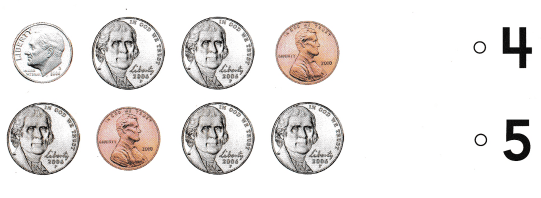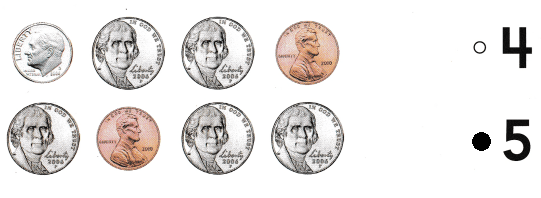# Texas Go Math Kindergarten Lesson 15.5 Answer Key Identify Coins

Refer to our Texas Go Math Kindergarten Answer Key Pdf to score good marks in the exams. Test yourself by practicing the problems from Texas Go Math Kindergarten Lesson 15.5 Answer Key Identify Coins.

## Texas Go Math Kindergarten Lesson 15.5 Answer Key Identify Coins

Essential Question
can you solve problems using the strategy act it out?

Unlock the ProblemExplanation:
There are 2 pennies circled in yellow
and 2 nickels circled in green, 3 dimes circled in red and 3 quarters circled in blue.

Directions
Circle the pennies in yellow. Circle the nickels in green. Circle the dimes in red. Circle the quarters in blue. Count and write the number of quarters.

Try Another Problem

Question 1.Question 2.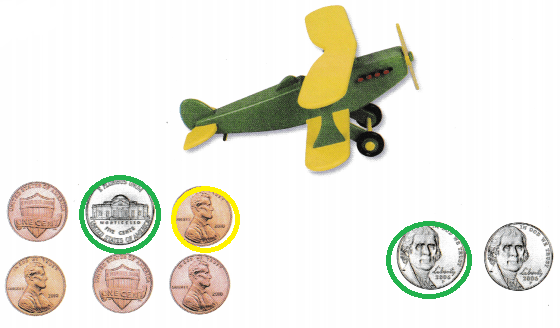Directions
1-2. Listen to the problem. Circle the set that shows the coins used to buy the item.

Share and Show

Question 3.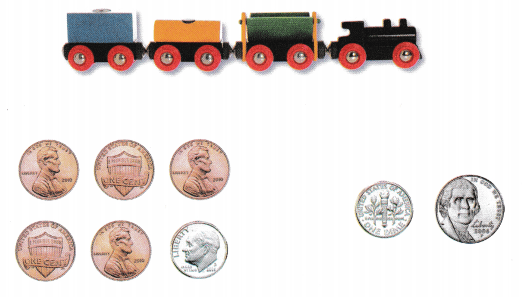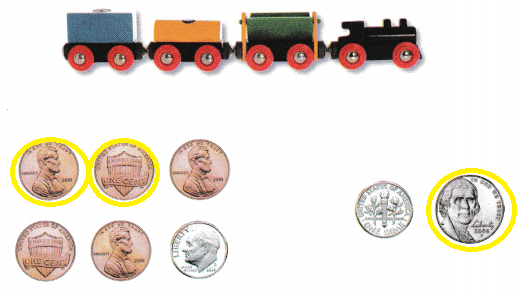Question 4.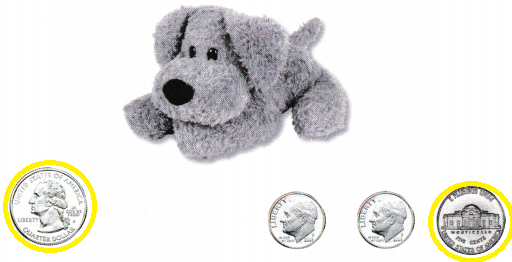0.25$+ 0.05$ = 0.30\$

Directions
3-4. Listen to the problem. Circle the set that shows the coins used to buy the item.

Home Activity

• Show your child a set of coins including a nickel, a dime, and a quarter. Have him or her tell you the name of each coin and describe how the coins are alike and how they are different.

Question 5.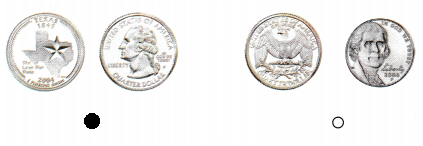Explanation:
In the first set we have 2 quarters
and in the second set we had 1 nickel and 1 quarter

Question 6.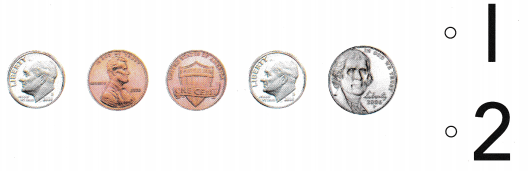Explanation:
There are 2 pennies in the group
marked the number 2

Question 7.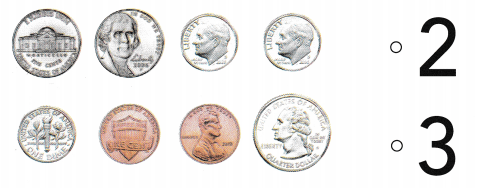Explanation:
Tina has these coins.
3 dimes that she have
So, marked the number 3

Directions
Choose the correct answer. 5. Which set has the some coins? 6. How many pennies are in this group? 7. Tina has these coins. How many dimes does she have?

### Texas Go Math Kindergarten Lesson 15.5 Homework and Practice

Question 1.Explanation:
Abe used four coins to buy a toy car
so, he used 3 nickels and 1 penny

Question 2.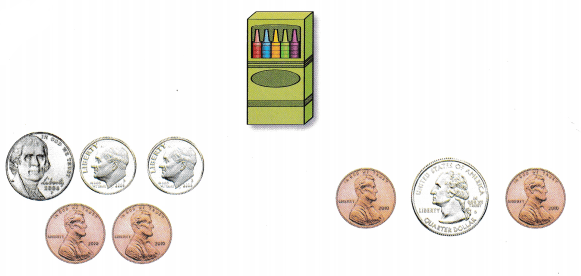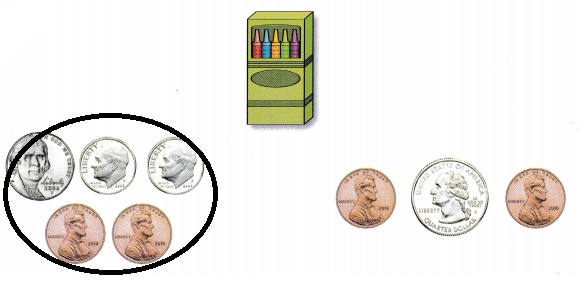Explanation:
Beth used five coins to buy a box of crayons.
so, he used 2 pennies 2 dimes and 1 nickel.

Directions
1. Abe used four coins to buy a toy car. Circle the set of coins Abe used to buy the car. 2. Beth used five coins to buy a box of crayons. Circle the set that shows the coins Beth used to buy the crayons.

Texas Test Prep

Question 3.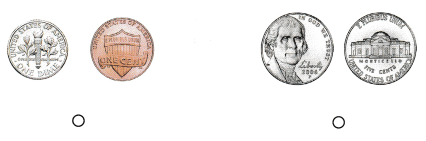Explanation:
The second set has the same coins they are 2 nickels
so, marked the second set

Question 4.Explanation:
There are 4 dimes in the above group
so, marked the number 4

Question 5.